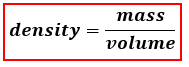# Problem: Osmium is one of the densest elements known. What is its density if 2.72 g has a volume of 0.121 cm3 ?

###### FREE Expert Solution

Density represents the mass of an object or compound within a given volume. When calculating for density, we use the following equation:79% (97 ratings)###### Problem Details

Osmium is one of the densest elements known. What is its density if 2.72 g has a volume of 0.121 cm3 ?